# The Mixed Integer Linear Programming Solver

### Example 7.3 Facility Location

Consider the classic facility location problem. Given a set L of customer locations and a set F of candidate facility sites, you must decide on which sites to build facilities and assign coverage of customer demand to these sites so as to minimize cost. All customer demand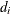must be satisfied, and each facility has a demand capacity limit C. The total cost is the sum of the distances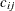between facility j and its assigned customer i, plus a fixed charge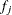for building a facility at site j. Let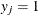represent choosing site j to build a facility, and 0 otherwise. Also, let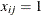represent the assignment of customer i to facility j, and 0 otherwise. This model can be formulated as the following integer linear program: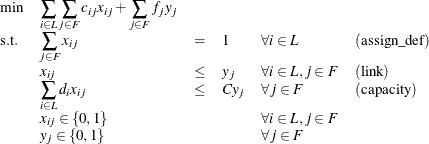Constraint (assign_def) ensures that each customer is assigned to exactly one site. Constraint (link) forces a facility to be built if any customer has been assigned to that facility. Finally, constraint (capacity) enforces the capacity limit at each site.

Consider also a variation of this same problem where there is no cost for building a facility. This problem is typically easier to solve than the original problem. For this variant, let the objective be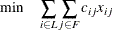First, construct a random instance of this problem by using the following DATA steps:

title 'Facility Location Problem';


%let NumCustomers  = 50;
%let NumSites      = 10;
%let SiteCapacity  = 35;
%let MaxDemand     = 10;
%let xmax          = 200;
%let ymax          = 100;
%let seed          = 938;

/* generate random customer locations */
data cdata(drop=i);
length name $8; do i = 1 to &NumCustomers; name = compress('C'||put(i,best.)); x = ranuni(&seed) * &xmax; y = ranuni(&seed) * &ymax; demand = ranuni(&seed) * &MaxDemand; output; end; run; /* generate random site locations and fixed charge */ data sdata(drop=i); length name$8;
do i = 1 to &NumSites;
name = compress('SITE'||put(i,best.));
x = ranuni(&seed) * &xmax;
y = ranuni(&seed) * &ymax;
fixed_charge = 30 * (abs(&xmax/2-x) + abs(&ymax/2-y));
output;
end;
run;


The following PROC OPTMODEL statements first generate and solve the model with the no-fixed-charge variant of the cost function. Next, they solve the fixed-charge model. Note that the solution to the model with no fixed charge is feasible for the fixed-charge model and should provide a good starting point for the MILP solver. Use the PRIMALIN option to provide an incumbent solution (warm start).


proc optmodel;
set <str> CUSTOMERS;
set <str> SITES init {};
/* x and y coordinates of CUSTOMERS and SITES */
num x {CUSTOMERS union SITES};
num y {CUSTOMERS union SITES};
num demand {CUSTOMERS};
num fixed_charge {SITES};

/* distance from customer i to site j */
num dist {i in CUSTOMERS, j in SITES}
= sqrt((x[i] - x[j])^2 + (y[i] - y[j])^2);

read data cdata into CUSTOMERS=[name] x y demand;
read data sdata into SITES=[name] x y fixed_charge;

var Assign {CUSTOMERS, SITES} binary;
var Build {SITES} binary;

min CostNoFixedCharge
= sum {i in CUSTOMERS, j in SITES} dist[i,j] * Assign[i,j];
min CostFixedCharge
= CostNoFixedCharge + sum {j in SITES} fixed_charge[j] * Build[j];

/* each customer assigned to exactly one site */
con assign_def {i in CUSTOMERS}:
sum {j in SITES} Assign[i,j] = 1;

/* if customer i assigned to site j, then facility must be built at j */
con link {i in CUSTOMERS, j in SITES}:
Assign[i,j] <= Build[j];

/* each site can handle at most &SiteCapacity demand */
con capacity {j in SITES}:
sum {i in CUSTOMERS} demand[i] * Assign[i,j] <=
&SiteCapacity * Build[j];

/* solve the MILP with no fixed charges */
solve obj CostNoFixedCharge with milp / logfreq = 500;

/* clean up the solution */
for {i in CUSTOMERS, j in SITES} Assign[i,j] = round(Assign[i,j]);
for {j in SITES} Build[j] = round(Build[j]);

call symput('varcostNo',put(CostNoFixedCharge,6.1));

/* create a data set for use by GPLOT */
create data CostNoFixedCharge_Data from
[customer site]={i in CUSTOMERS, j in SITES: Assign[i,j] = 1}
xi=x[i] yi=y[i] xj=x[j] yj=y[j];

/* solve the MILP, with fixed charges with warm start */
solve obj CostFixedCharge with milp / primalin logfreq = 500;

/* clean up the solution */
for {i in CUSTOMERS, j in SITES} Assign[i,j] = round(Assign[i,j]);
for {j in SITES} Build[j] = round(Build[j]);

num varcost = sum {i in CUSTOMERS, j in SITES} dist[i,j] * Assign[i,j].sol;
num fixcost = sum {j in SITES} fixed_charge[j] * Build[j].sol;
call symput('varcost', put(varcost,6.1));
call symput('fixcost', put(fixcost,5.1));
call symput('totalcost', put(CostFixedCharge,6.1));

/* create a data set for use by GPLOT */
create data CostFixedCharge_Data from
[customer site]={i in CUSTOMERS, j in SITES: Assign[i,j] = 1}
xi=x[i] yi=y[i] xj=x[j] yj=y[j];
quit;



The information printed in the log for the no-fixed-charge model is displayed in Output 7.3.1.

Output 7.3.1: OPTMODEL Log for Facility Location with No Fixed Charges

 NOTE: Problem generation will use 4 threads. NOTE: The problem has 510 variables (0 free, 0 fixed). NOTE: The problem has 510 binary and 0 integer variables. NOTE: The problem has 560 linear constraints (510 LE, 50 EQ, 0 GE, 0 range). NOTE: The problem has 2010 linear constraint coefficients. NOTE: The problem has 0 nonlinear constraints (0 LE, 0 EQ, 0 GE, 0 range). NOTE: The MILP presolver value AUTOMATIC is applied. NOTE: The MILP presolver removed 10 variables and 500 constraints. NOTE: The MILP presolver removed 1010 constraint coefficients. NOTE: The MILP presolver modified 0 constraint coefficients. NOTE: The presolved problem has 500 variables, 60 constraints, and 1000 constraint coefficients. NOTE: The MILP solver is called. Node  Active    Sols    BestInteger      BestBound      Gap    Time 0       1       2    972.1737321              0    972.2       0 0       1       2    972.1737321    961.2403449    1.14%       0 0       1       2    972.1737321    966.4828342    0.59%       0 0       1       3    966.4832160    966.4828342    0.00%       0 NOTE: The MILP solver added 3 cuts with 102 cut coefficients at the root. NOTE: Optimal within relative gap. NOTE: Objective = 966.48321599.

The results from the warm start approach are shown in Output 7.3.2.

Output 7.3.2: OPTMODEL Log for Facility Location with Fixed Charges, Using Warm Start

 NOTE: Problem generation will use 4 threads. NOTE: The problem has 510 variables (0 free, 0 fixed). NOTE: The problem uses 1 implicit variables. NOTE: The problem has 510 binary and 0 integer variables. NOTE: The problem has 560 linear constraints (510 LE, 50 EQ, 0 GE, 0 range). NOTE: The problem has 2010 linear constraint coefficients. NOTE: The problem has 0 nonlinear constraints (0 LE, 0 EQ, 0 GE, 0 range). NOTE: The MILP presolver value AUTOMATIC is applied. NOTE: The MILP presolver removed 0 variables and 0 constraints. NOTE: The MILP presolver removed 0 constraint coefficients. NOTE: The MILP presolver modified 0 constraint coefficients. NOTE: The presolved problem has 510 variables, 560 constraints, and 2010 constraint coefficients. NOTE: The MILP solver is called. Node  Active    Sols    BestInteger      BestBound      Gap    Time 0       1       3  16070.0150023              0    16070       0 0       1       3  16070.0150023   9946.2514269   61.57%       0 0       1       3  16070.0150023   9965.3660728   61.26%       0 0       1       3  16070.0150023   9992.5098441   60.82%       1 0       1       3  16070.0150023  10008.1168033   60.57%       1 0       1       5  12714.8421527  10015.7276884   26.95%       1 0       1       5  12714.8421527  10022.7765711   26.86%       2 0       1       6  12697.5037984  10031.4554268   26.58%       2 0       1       6  12697.5037984  10038.3630945   26.49%       2 0       1       6  12697.5037984  10040.1836986   26.47%       3 0       1       7  12681.6662283  10041.2236063   26.30%       3 0       1       7  12681.6662283  10041.8276236   26.29%       3 0       1       7  12681.6662283  10042.4192876   26.28%       3 0       1       7  12681.6662283  10042.4192876   26.28%       6 NOTE: The MILP solver added 30 cuts with 862 cut coefficients at the root. 12      10      10  10982.8321843  10050.5594169    9.28%       7 113      15      11  10962.4644900  10940.8960590    0.20%      11 141      16      12  10948.4603467  10942.5795938    0.05%      12 173      17      13  10948.4603465  10946.1080596    0.02%      12 181       9      13  10948.4603465  10947.6003284    0.01%      12 NOTE: Optimal within relative gap. NOTE: Objective = 10948.460346.

The following two SAS programs produce a plot of the solutions for both variants of the model, using data sets produced by PROC OPTMODEL:

title1 h=1.5 "Facility Location Problem";
title2 "TotalCost = &varcostNo (Variable = &varcostNo, Fixed = 0)";

data csdata;
set cdata(rename=(y=cy)) sdata(rename=(y=sy));
run;

/* create Annotate data set to draw line between customer and assigned site */
%annomac;
data anno(drop=xi yi xj yj);
%SYSTEM(2, 2, 2);
set CostNoFixedCharge_Data(keep=xi yi xj yj);
%LINE(xi, yi, xj, yj, *, 1, 1);
run;

proc gplot data=csdata anno=anno;
axis1 label=none order=(0 to &xmax by 10);
axis2 label=none order=(0 to &ymax by 10);
symbol1 value=dot interpol=none
pointlabel=("#name" nodropcollisions height=1) cv=black;
symbol2 value=diamond interpol=none
pointlabel=("#name" nodropcollisions color=blue height=1) cv=blue;
plot cy*x sy*x / overlay haxis=axis1 vaxis=axis2;
run;
quit;



The output of the first program is shown in Output 7.3.3.

Output 7.3.3: Solution Plot for Facility Location with No Fixed Charges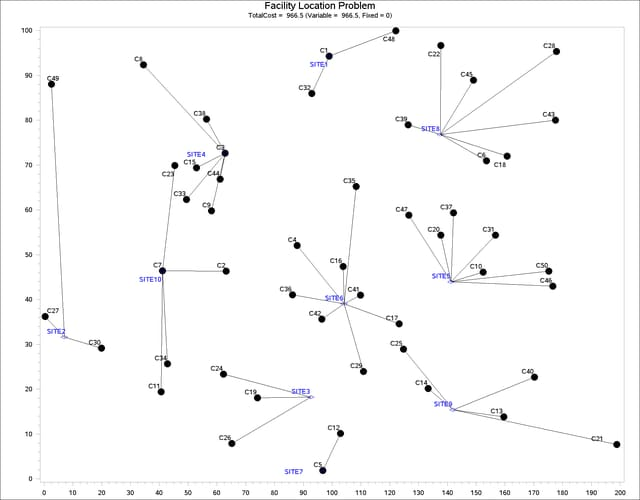The output of the second program is shown in Output 7.3.4.

title1 "Facility Location Problem";
title2 "TotalCost = &totalcost (Variable = &varcost, Fixed = &fixcost)";

/* create Annotate data set to draw line between customer and assigned site */
data anno(drop=xi yi xj yj);
%SYSTEM(2, 2, 2);
set CostFixedCharge_Data(keep=xi yi xj yj);
%LINE(xi, yi, xj, yj, *, 1, 1);
run;

proc gplot data=csdata anno=anno;
axis1 label=none order=(0 to &xmax by 10);
axis2 label=none order=(0 to &ymax by 10);
symbol1 value=dot interpol=none
pointlabel=("#name" nodropcollisions height=1) cv=black;
symbol2 value=diamond interpol=none
pointlabel=("#name" nodropcollisions color=blue height=1) cv=blue;
plot cy*x sy*x / overlay haxis=axis1 vaxis=axis2;
run;
quit;


Output 7.3.4: Solution Plot for Facility Location with Fixed Charges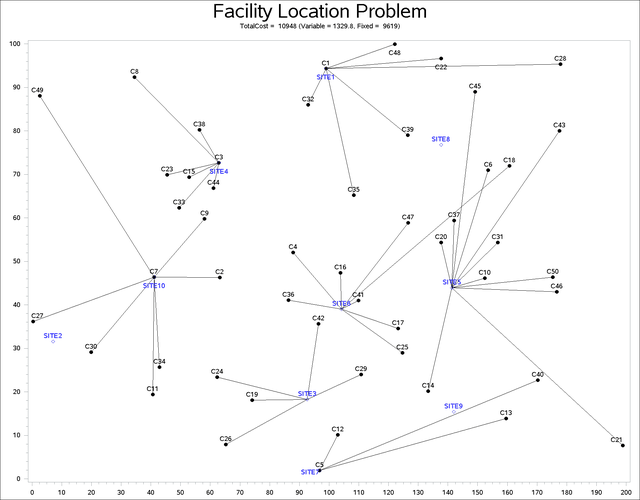The economic trade-off for the fixed-charge model forces you to build fewer sites and push more demand to each site.

It is possible to expedite the solution of the fixed-charge facility location problem by choosing appropriate branching priorities for the decision variables. Recall that for each site j, the value of the variable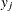determines whether or not a facility is built on that site. Suppose you decide to branch on the variablesbefore the variables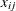. You can set a higher branching priority forby using the .priority suffix for the Build variables in PROC OPTMODEL, as follows:

   for{j in SITES} Build[j].priority=10;


Setting higher branching priorities for certain variables is not guaranteed to speed up the MILP solver, but it can be helpful in some instances. The following program creates and solves an instance of the facility location problem, giving higher priority to the variables. The LOGFREQ= option is used to abbreviate the node log.


%let NumCustomers  = 45;
%let NumSites      = 8;
%let SiteCapacity  = 35;
%let MaxDemand     = 10;
%let xmax          = 200;
%let ymax          = 100;
%let seed          = 2345;

/* generate random customer locations */
data cdata(drop=i);
length name $8; do i = 1 to &NumCustomers; name = compress('C'||put(i,best.)); x = ranuni(&seed) * &xmax; y = ranuni(&seed) * &ymax; demand = ranuni(&seed) * &MaxDemand; output; end; run; /* generate random site locations and fixed charge */ data sdata(drop=i); length name$8;
do i = 1 to &NumSites;
name = compress('SITE'||put(i,best.));
x = ranuni(&seed) * &xmax;
y = ranuni(&seed) * &ymax;
fixed_charge = (abs(&xmax/2-x) + abs(&ymax/2-y)) / 2;
output;
end;
run;

proc optmodel;
set <str> CUSTOMERS;
set <str> SITES init {};

/* x and y coordinates of CUSTOMERS and SITES */
num x {CUSTOMERS union SITES};
num y {CUSTOMERS union SITES};
num demand {CUSTOMERS};
num fixed_charge {SITES};

/* distance from customer i to site j */
num dist {i in CUSTOMERS, j in SITES}
= sqrt((x[i] - x[j])^2 + (y[i] - y[j])^2);

read data cdata into CUSTOMERS=[name] x y demand;
read data sdata into SITES=[name] x y fixed_charge;

var Assign {CUSTOMERS, SITES} binary;
var Build {SITES} binary;

min CostFixedCharge
= sum {i in CUSTOMERS, j in SITES} dist[i,j] * Assign[i,j]
+ sum {j in SITES} fixed_charge[j] * Build[j];

/* each customer assigned to exactly one site */
con assign_def {i in CUSTOMERS}:
sum {j in SITES} Assign[i,j] = 1;

/* if customer i assigned to site j, then facility must be built at j */
con link {i in CUSTOMERS, j in SITES}:
Assign[i,j] <= Build[j];

/* each site can handle at most &SiteCapacity demand */
con capacity {j in SITES}:
sum {i in CUSTOMERS} demand[i] * Assign[i,j] <= &SiteCapacity * Build[j];

/* assign priority to Build variables (y) */
for{j in SITES} Build[j].priority=10;

/* solve the MILP with fixed charges, using branching priorities */
solve obj CostFixedCharge with milp / logfreq=1000;
quit;


The resulting output is shown in Output 7.3.5.

Output 7.3.5: PROC OPTMODEL Log for Facility Location with Branching Priorities

 NOTE: There were 45 observations read from the data set WORK.CDATA. NOTE: There were 8 observations read from the data set WORK.SDATA. NOTE: Problem generation will use 4 threads. NOTE: The problem has 368 variables (0 free, 0 fixed). NOTE: The problem has 368 binary and 0 integer variables. NOTE: The problem has 413 linear constraints (368 LE, 45 EQ, 0 GE, 0 range). NOTE: The problem has 1448 linear constraint coefficients. NOTE: The problem has 0 nonlinear constraints (0 LE, 0 EQ, 0 GE, 0 range). NOTE: The MILP presolver value AUTOMATIC is applied. NOTE: The MILP presolver removed 0 variables and 0 constraints. NOTE: The MILP presolver removed 0 constraint coefficients. NOTE: The MILP presolver modified 0 constraint coefficients. NOTE: The presolved problem has 368 variables, 413 constraints, and 1448 constraint coefficients. NOTE: The MILP solver is called. Node  Active    Sols    BestInteger      BestBound      Gap    Time 0       1       3   2823.1827978              0   2823.2       0 0       1       3   2823.1827978   1727.0208789   63.47%       0 0       1       3   2823.1827978   1752.0649013   61.13%       0 0       1       3   2823.1827978   1764.4145721   60.01%       0 0       1       3   2823.1827978   1771.3542742   59.38%       1 0       1       3   2823.1827978   1778.1649446   58.77%       1 0       1       3   2823.1827978   1779.9319639   58.61%       1 0       1       3   2823.1827978   1780.4398220   58.57%       1 0       1       6   1903.7976887   1781.4348074    6.87%       2 0       1       6   1903.7976887   1781.6818875    6.85%       2 0       1       6   1903.7976887   1781.9825516    6.84%       2 0       1       6   1903.7976887   1784.3735066    6.69%       2 0       1       6   1903.7976887   1785.1171912    6.65%       3 0       1       6   1903.7976887   1785.1318270    6.65%       3 0       1       6   1903.7976887   1785.1691077    6.65%       3 0       1       6   1903.7976887   1785.2000207    6.64%       3 0       1       6   1903.7976887   1785.2000207    6.64%       3 NOTE: The MILP solver added 28 cuts with 767 cut coefficients at the root. 4       1       8   1852.5588919   1802.5216297    2.78%       4 52      46      10   1835.0251598   1804.8458565    1.67%       5 244     215      11   1834.9126635   1808.0082480    1.49%       7 296     245      12   1830.7850312   1809.1809114    1.19%       8 523     282      13   1825.1666003   1812.4410890    0.70%      11 608     293      14   1825.1666001   1814.3493473    0.60%      13 642     135      15   1821.5115363   1815.4351037    0.33%      13 691      46      16   1819.9124342   1818.1426710    0.10%      13 735       4      16   1819.9124342   1819.7466770    0.01%      14 NOTE: Optimal within relative gap. NOTE: Objective = 1819.9124342.